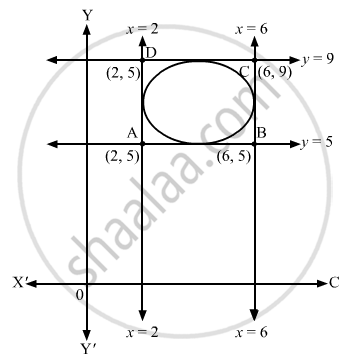Advertisement Remove all ads

# Write the Coordinates of the Centre of the Circle Inscribed in the Square Formed by the Lines X = 2, X = 6, Y = 5 and Y = 9. - Mathematics

Write the coordinates of the centre of the circle inscribed in the square formed by the lines x = 2, x = 6, y = 5 and y = 9.

Advertisement Remove all ads

#### SolutionFrom the figure, we can see that the vertices of the square are (2, 5), (6, 5), (2, 9) and (6, 9).
The vertices of the diagonals are (2, 9), (6, 5) and (2, 5),(6, 9).
∴ Coordinates of the centre = $\left( \frac{6 + 2}{2}, \frac{5 + 9}{2} \right) = \left( 4, 7 \right)$

Is there an error in this question or solution?
Advertisement Remove all ads

#### APPEARS IN

RD Sharma Class 11 Mathematics Textbook
Chapter 24 The circle
Q 9 | Page 38
Advertisement Remove all ads

#### Video TutorialsVIEW ALL 

Advertisement Remove all ads
Share
Notifications

View all notifications

Forgot password?